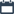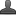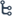## Thursday, September 24, 2020

•September 24, 2020
••, ,
•3 comments

## Mathematics trigonometry Exercise - 11.1

Hi friends and my dear students! In this post, I have covered Andhra Pradesh SSC(10th class) Mathematics trigonometry Exercise - 11.1 Solutions. After Reading Mathematics trigonometry Exercise, Please do share it with your friends. You can Learn Maths for All Classes here.

1. In right angle triangle ABC, 8 cm, 15 cm and 17 cm are the lengths of AB,BC and CA respectively. Then, find out sin A, Cos A, and tan A.

Sol: Given in right angle triangle ABC, 8 Cm, 15 Cm and 17 Cm are the length of AB, BC and CA respectively

i) sin A= Opposite side /Hyptenuse =BC/AC=15/17

ii) Cos A = Adjacent side/Hyptenuse = AB/AC =8/17

iii) Tan A =Opposite side/Adjacent side=BC/AB=15/8

2. The sides of a Right angle triangle PQR are PQ = 7 cm, QR = 24 cm and √Q = 90 respectively. Then find, tan P, tan R.

Sol:  Given the sides of a right angle triangle PQR are PQ = 7 cm, QR = 24 cm and Q=90

BY Pythagoras theorem

PR 2 = PQ 2+ QR 2

PR 2= (7) 2+ (25) 2

PR 2= 49+ 576

PR 2= 625

PR =√625

PR =25

Tan R= QP/QR=7/24

Tan p -Tan R = 24/7 - 7/24

=  24 × 24 - 7 × 7

-----------------

168

= 527/168

Introduction To Trigonometry

3. In a right angle triangle ABC with right angle at B, in which a= 24 units, b = 25 units andBAC =Ø Then, find CosØ and tanØ.

Sol: Given in a right angle triangle ABC with right angle at B, in which

a = 24 units, b= 25 units and BAC=Ø

BY Pythagoras theorem

AC 2 = BC 2+ AB 2

(25) 2= (24) 2+ (AB) 2

625 = 576 + (AB) 2

(AB) 2= 625-576

(AB) 2 =49

(AB)  = √49

AB = 7

I ) Cos Ø= AB/AC = 7/ 25

ii) Tan Ø =BC/AB = 24/7

4. If Cos A =12/13 than find sin A tan A.

Sol: Given Cos A = Adjacent side/Hypotenuse=12/13

BY Pythagoras theorem

AC 2 = BC 2 + AB 2

13 =12 2+ BC 2

BC 2 =13 2-12 2

BC 2 =169-144

BC=√25

BC=√5×5

BC=5

Now

Sin A = BC/AC =5/13

Tan A = BC/AB =5/12

5. If 3 tan A = 4 than find sin A Cos A

Sol: Given tan A = Opposite side/Adjacent side= 4/3

BY Pythagoras theorem

AC 2= AB 2 + BC 2

AC 2= 32+42

AC 2=9+16

AC 2=25

AC=√25

AC=5

Now    Sin A = BC/AC =4/5

Cos A = AC/AB =3/5

1.This comment has been removed by the author.

2.Hi Shaik, Many students who are good at maths, even they hate math theorems. They find it challenging to prove and struggle to remember the steps required to solve. Only good math tutors can make students understand the derivations of theorems and keep them motivated in Math tuition. Theorems not only help to solve mathematical problems easily but their proofs also help to develop a deeper understanding of the underlying concepts. You shared a large no of mathematical theorems and related problems here. Thanks for sharing such useful content.

3.Your blog took to me an out and out basic spot. It is an important and evident article to redesign data. Appreciation for sharing an article like this.Tutoring Services In Hutto